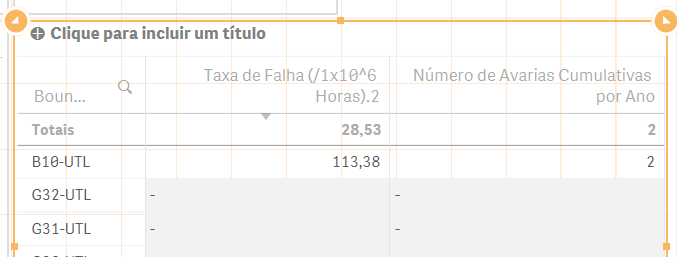# New to Qlik Sense

Discussion board where members can get started with Qlik Sense.

Highlighted
New Contributor II

## Getting the value I want on the Totals of a Table but not on the right row

Hi,

I have the following table on Qlik Sense:To calculate "Número de Avarias Cumulativas por Ano" I have this formula:

Aggr(Count(Ordem), [Equipamento-Num. SAP])

For "Taxa de Falha" I have this:

Aggr(Count(Ordem), [Equipamento-Num. SAP])/((Max({<Factory=, Unidade=, [Nome Equipamento]=, [TAG.Nr]=, Boundary=>}(Data))-MakeDate(2008,01,01))*24/pow(10,6))

I have to use {<Factory=, Unidade=, [Nome Equipamento]=, [TAG.Nr]=, Boundary=>} to avoid filters from removing the latest date, since I have no records for the last day I am filtering.

The value I must have for B10-UTL is 28,53. The value that appears on the Totals. Why cannot I have that value for B10-UTL?

Thank you for your answers.

Best regards,

Tags (4)
2 Replies
New Contributor II

## Re: Getting the value I want on the Totals of a Table but not on the right row

[Update]

This has to do with the fact that the value of Max({<Factory=, Unidade=, [Nome Equipamento]=, [TAG.Nr]=, Boundary=>}Data) changes for every Boundary (first Column) in the table. Is there a way to fix the value unchangeable for each row?

This probably has to do with the structure and connections I have between the tables I uploaded and the value of Data I select is removed because it has no Boundary attributed to it.

New Contributor II

## Re: Getting the value I want on the Totals of a Table but not on the right row

[Update]

Was a rookie mistake. Started working once I changed to:

Aggr(Count(Ordem), [Equipamento-Num. SAP])/(((Max({<Factory=, Unidade=, [Nome Equipamento]=, [TAG.Nr]=, Boundary=>} Total Data)-MakeDate(2008, 01, 01))*24/pow(10,6)))